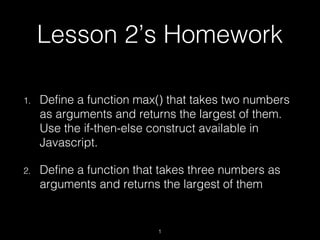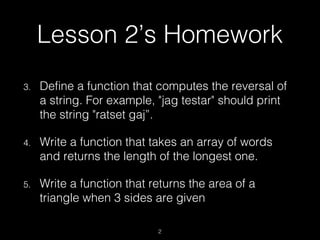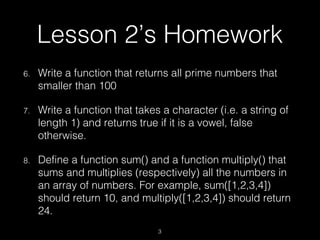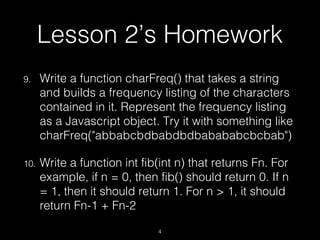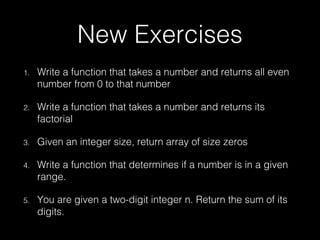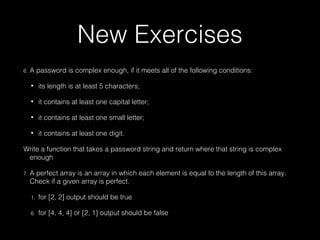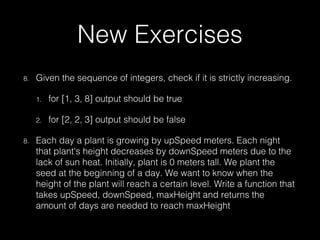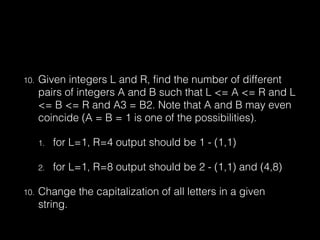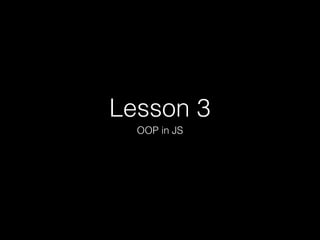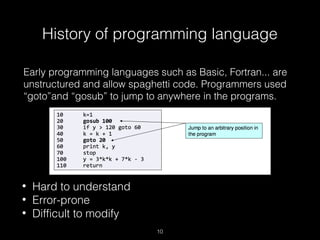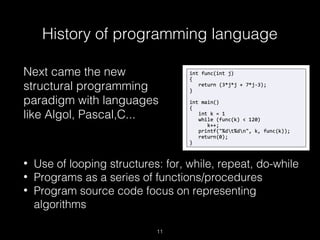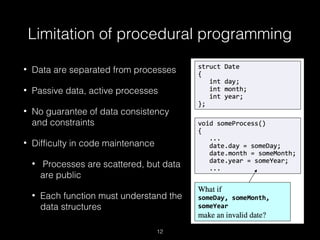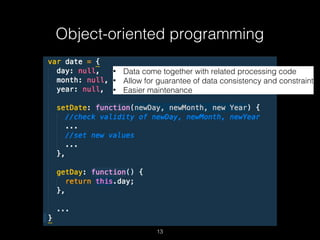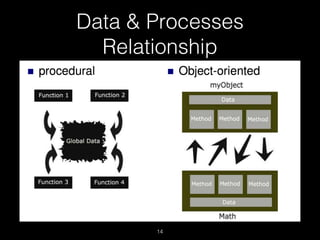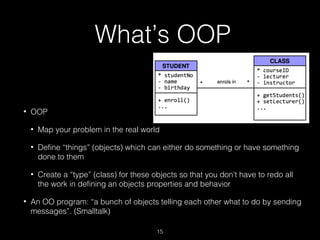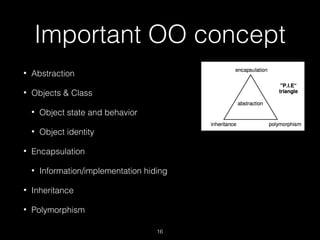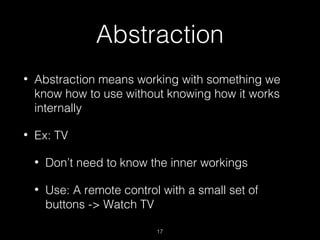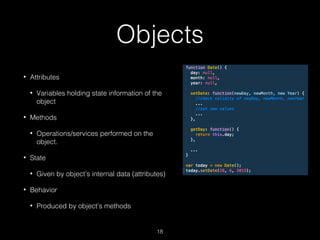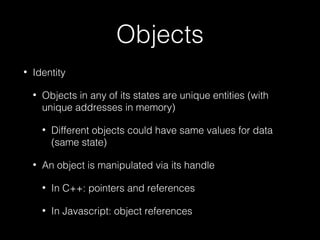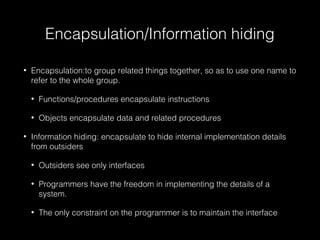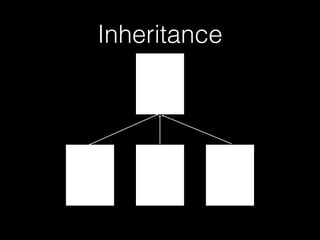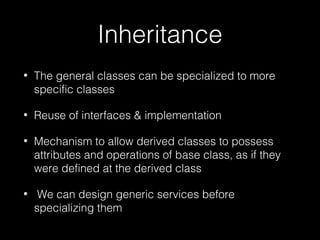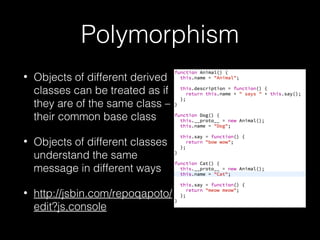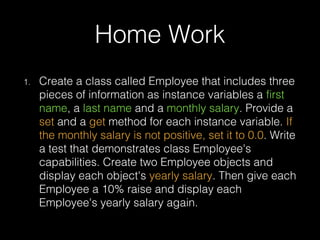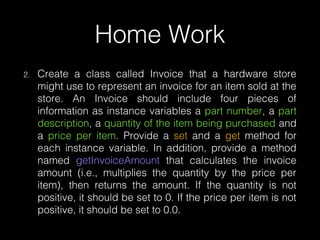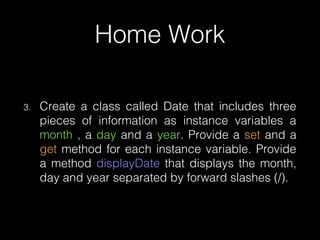1 de 26

### Hub102 - JS - Lesson3

1. Lesson 2’s Homework 1. Define a function max() that takes two numbers as arguments and returns the largest of them. Use the if-then-else construct available in Javascript. 2. Define a function that takes three numbers as arguments and returns the largest of them 1
2. 3. Define a function that computes the reversal of a string. For example, "jag testar" should print the string "ratset gaj”. 4. Write a function that takes an array of words and returns the length of the longest one. 5. Write a function that returns the area of a triangle when 3 sides are given Lesson 2’s Homework 2
3. 6. Write a function that returns all prime numbers that smaller than 100 7. Write a function that takes a character (i.e. a string of length 1) and returns true if it is a vowel, false otherwise. 8. Define a function sum() and a function multiply() that sums and multiplies (respectively) all the numbers in an array of numbers. For example, sum([1,2,3,4]) should return 10, and multiply([1,2,3,4]) should return 24. Lesson 2’s Homework 3
4. 9. Write a function charFreq() that takes a string and builds a frequency listing of the characters contained in it. Represent the frequency listing as a Javascript object. Try it with something like charFreq("abbabcbdbabdbdbabababcbcbab") 10. Write a function int fib(int n) that returns Fn. For example, if n = 0, then fib() should return 0. If n = 1, then it should return 1. For n > 1, it should return Fn-1 + Fn-2 Lesson 2’s Homework 4
5. New Exercises 1. Write a function that takes a number and returns all even number from 0 to that number 2. Write a function that takes a number and returns its factorial 3. Given an integer size, return array of size zeros 4. Write a function that determines if a number is in a given range. 5. You are given a two-digit integer n. Return the sum of its digits.
6. New Exercises 6. A password is complex enough, if it meets all of the following conditions: • its length is at least 5 characters; • it contains at least one capital letter; • it contains at least one small letter; • it contains at least one digit. Write a function that takes a password string and return where that string is complex enough 7. A perfect array is an array in which each element is equal to the length of this array. Check if a given array is perfect. 1. for [2, 2] output should be true 6. for [4, 4, 4] or [2, 1] output should be false
7. New Exercises 8. Given the sequence of integers, check if it is strictly increasing. 1. for [1, 3, 8] output should be true 2. for [2, 2, 3] output should be false 8. Each day a plant is growing by upSpeed meters. Each night that plant's height decreases by downSpeed meters due to the lack of sun heat. Initially, plant is 0 meters tall. We plant the seed at the beginning of a day. We want to know when the height of the plant will reach a certain level. Write a function that takes upSpeed, downSpeed, maxHeight and returns the amount of days are needed to reach maxHeight
8. 10. Given integers L and R, find the number of different pairs of integers A and B such that L <= A <= R and L <= B <= R and A3 = B2. Note that A and B may even coincide (A = B = 1 is one of the possibilities). 1. for L=1, R=4 output should be 1 - (1,1) 2. for L=1, R=8 output should be 2 - (1,1) and (4,8) 10. Change the capitalization of all letters in a given string.
9. Lesson 3 OOP in JS
10. History of programming language Early programming languages such as Basic, Fortran... are unstructured and allow spaghetti code. Programmers used “goto”and “gosub” to jump to anywhere in the programs. • Hard to understand • Error-prone • Difficult to modify 10
11. Next came the new structural programming paradigm with languages like Algol, Pascal,C... History of programming language • Use of looping structures: for, while, repeat, do-while • Programs as a series of functions/procedures • Program source code focus on representing algorithms 11
12. Limitation of procedural programming • Data are separated from processes • Passive data, active processes • No guarantee of data consistency and constraints • Difficulty in code maintenance • Processes are scattered, but data are public • Each function must understand the data structures 12
13. Object-oriented programming • Data come together with related processing code • Allow for guarantee of data consistency and constraint • Easier maintenance 13
14. Data & Processes Relationship 14
15. What’s OOP • OOP • Map your problem in the real world • Define “things” (objects) which can either do something or have something done to them • Create a “type” (class) for these objects so that you don’t have to redo all the work in defining an objects properties and behavior • An OO program: “a bunch of objects telling each other what to do by sending messages”. (Smalltalk) 15
16. Important OO concept • Abstraction • Objects & Class • Object state and behavior • Object identity • Encapsulation • Information/implementation hiding • Inheritance • Polymorphism 16
17. Abstraction • Abstraction means working with something we know how to use without knowing how it works internally • Ex: TV • Don’t need to know the inner workings • Use: A remote control with a small set of buttons -> Watch TV 17
18. Objects • Attributes • Variables holding state information of the object • Methods • Operations/services performed on the object. • State • Given by object’s internal data (attributes) • Behavior • Produced by object’s methods 18
19. Objects • Identity • Objects in any of its states are unique entities (with unique addresses in memory) • Different objects could have same values for data (same state) • An object is manipulated via its handle • In C++: pointers and references • In Javascript: object references
20. Encapsulation/Information hiding • Encapsulation:to group related things together, so as to use one name to refer to the whole group. • Functions/procedures encapsulate instructions • Objects encapsulate data and related procedures • Information hiding: encapsulate to hide internal implementation details from outsiders • Outsiders see only interfaces • Programmers have the freedom in implementing the details of a system. • The only constraint on the programmer is to maintain the interface
21. Inheritance Animal name •eat() •breathe() Duck name •swim() •fly() Dog name •bark() •run() Coral name
22. Inheritance • The general classes can be specialized to more specific classes • Reuse of interfaces & implementation • Mechanism to allow derived classes to possess attributes and operations of base class, as if they were defined at the derived class • We can design generic services before specializing them
23. Polymorphism • Objects of different derived classes can be treated as if they are of the same class – their common base class • Objects of different classes understand the same message in different ways • http://jsbin.com/repoqapoto/ edit?js,console
24. Home Work 1. Create a class called Employee that includes three pieces of information as instance variables a first name, a last name and a monthly salary. Provide a set and a get method for each instance variable. If the monthly salary is not positive, set it to 0.0. Write a test that demonstrates class Employee's capabilities. Create two Employee objects and display each object's yearly salary. Then give each Employee a 10% raise and display each Employee's yearly salary again.
25. Home Work 2. Create a class called Invoice that a hardware store might use to represent an invoice for an item sold at the store. An Invoice should include four pieces of information as instance variables a part number, a part description, a quantity of the item being purchased and a price per item. Provide a set and a get method for each instance variable. In addition, provide a method named getInvoiceAmount that calculates the invoice amount (i.e., multiplies the quantity by the price per item), then returns the amount. If the quantity is not positive, it should be set to 0. If the price per item is not positive, it should be set to 0.0.
26. Home Work 3. Create a class called Date that includes three pieces of information as instance variables a month , a day and a year. Provide a set and a get method for each instance variable. Provide a method displayDate that displays the month, day and year separated by forward slashes (/).